Introduction to dendextend

2021-10-27

Author: Tal Galili ( Tal.Galili@gmail.com )

tl;dr: the dendextend package let’s you create figures like this: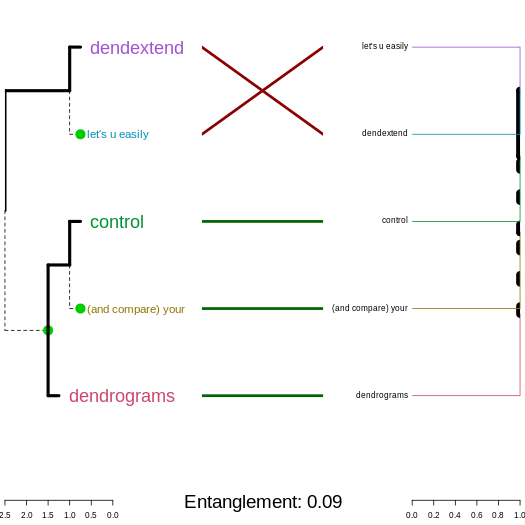Introduction

The dendextend package offers a set of functions for extending dendrogram objects in R, letting you visualize and compare trees of hierarchical clusterings, you can:

• Adjust a tree’s graphical parameters - the color, size, type, etc of its branches, nodes and labels.
• Visually and statistically compare different dendrograms to one another.

The goal of this document is to introduce you to the basic functions that dendextend provides, and show how they may be applied. We will make extensive use of “chaining” (explained next).

Prerequisites

Acknowledgement

This package was made possible by the the support of my thesis adviser Yoav Benjamini, as well as code contributions from many R users. They are:

#>   "Tal Galili <tal.galili@gmail.com> [aut, cre, cph] (https://www.r-statistics.com)"
#>   "Gavin Simpson [ctb]"
#>   "Gregory Jefferis <jefferis@gmail.com> [ctb] (imported code from his dendroextras package)"
#>   "Marco Gallotta [ctb] (a.k.a: marcog)"
#>   "Johan Renaudie [ctb] (https://github.com/plannapus)"
#>   "R core team [ctb] (Thanks for the Infastructure, and code in the examples)"
#>   "Kurt Hornik [ctb]"
#>   "Uwe Ligges [ctb]"
#>   "Andrej-Nikolai Spiess [ctb]"
#>  "Steve Horvath <SHorvath@mednet.ucla.edu> [ctb]"
#>  "Peter Langfelder <Peter.Langfelder@gmail.com> [ctb]"
#>  "skullkey [ctb]"
#>  "Mark Van Der Loo <mark.vanderloo@gmail.com> [ctb] (https://github.com/markvanderloo d3dendrogram)"
#>  "Yoav Benjamini [ths]"

The design of the dendextend package (and this manual!) is heavily inspired by Hadley Wickham’s work. Especially his text on writing an R package, the devtools package, and the dplyr package (specifically the use of chaining, and the Introduction text to dplyr).

Chaining

Function calls in dendextend often get a dendrogram and returns a (modified) dendrogram. This doesn’t lead to particularly elegant code if you want to do many operations at once. The same is true even in the first stage of creating a dendrogram.

In order to construct a dendrogram, you will (often) need to go through several steps. You can either do so while keeping the intermediate results:

d1 <- c(1:5) # some data
d2 <- dist(d1)
d3 <- hclust(d2, method = "average")
dend <- as.dendrogram(d3)

Or, you can also wrap the function calls inside each other:

dend <- as.dendrogram(hclust(dist(c(1:5)), method = "average"))

However, both solutions are not ideal: the first solution includes redundant intermediate objects, while the second is difficult to read (since the order of the operations is from inside to out, while the arguments are a long way away from the function).

To get around this problem, dendextend encourages the use of the %>% (“pipe” or “chaining”) operator (imported from the magrittr package). This turns x %>% f(y) into f(x, y) so you can use it to rewrite (“chain”) multiple operations such that they can be read from left-to-right, top-to-bottom.

For example, the following will be written as it would be explained:

dend <- c(1:5) %>% # take the a vector from 1 to 5
dist %>% # calculate a distance matrix,
hclust(method = "average") %>% # on it compute hierarchical clustering using the "average" method,
as.dendrogram # and lastly, turn that object into a dendrogram.

For more details, you may look at:

A dendrogram is a nested list of lists with attributes

The first step is working with dendrograms, is to understand that they are just a nested list of lists with attributes. Let us explore this for the following (tiny) tree:

# Create a dend:
dend <- 1:2 %>% dist %>% hclust %>% as.dendrogram
# and plot it:
dend %>% plot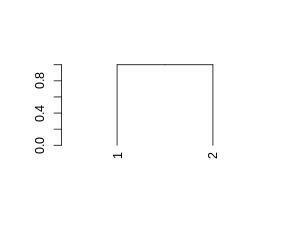And here is its structure (a nested list of lists with attributes):

dend %>% unclass %>% str
#> List of 2
#>  \$ : int 1
#>   ..- attr(*, "label")= int 1
#>   ..- attr(*, "members")= int 1
#>   ..- attr(*, "height")= num 0
#>   ..- attr(*, "leaf")= logi TRUE
#>  \$ : int 2
#>   ..- attr(*, "label")= int 2
#>   ..- attr(*, "members")= int 1
#>   ..- attr(*, "height")= num 0
#>   ..- attr(*, "leaf")= logi TRUE
#>  - attr(*, "members")= int 2
#>  - attr(*, "midpoint")= num 0.5
#>  - attr(*, "height")= num 1
dend %>% class
#>  "dendrogram"

Installation

To install the stable version on CRAN use:

install.packages('dendextend')

To install the GitHub version:

require2 <- function (package, ...) {
if (!require(package)) install.packages(package); library(package)
}

## require2('installr')
## install.Rtools() # run this if you are using Windows and don't have Rtools installed

require2("devtools")
devtools::install_github('talgalili/dendextend')
<!-- require2("Rcpp") -->

# Having colorspace is also useful, since it is used
# In various examples in the vignettes
require2("colorspace")

And then you may load the package using:

library(dendextend)

How to explore a dendrogram’s parameters

Taking a first look at a dendrogram

For the following simple tree:

# Create a dend:
dend <- 1:5 %>% dist %>% hclust %>% as.dendrogram
# Plot it:
dend %>% plot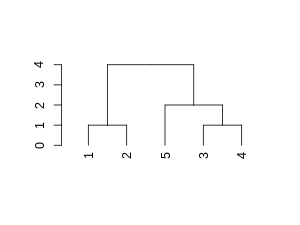Here are some basic parameters we can get:

dend %>% labels # get the labels of the tree
#>  1 2 5 3 4
dend %>% nleaves # get the number of leaves of the tree
#>  5
dend %>% nnodes # get the number of nodes in the tree (including leaves)
#>  9
#> --[dendrogram w/ 2 branches and 5 members at h = 4]
#>   |--[dendrogram w/ 2 branches and 2 members at h = 1]
#>   |  |--leaf 1
#>   |  `--leaf 2
#>   `--[dendrogram w/ 2 branches and 3 members at h = 2]
#>      |--leaf 5
#>      `--[dendrogram w/ 2 branches and 2 members at h = 1]
#>         |--leaf 3
#>         `--leaf 4
#> etc...

Next let us look at more sophisticated outputs.

How to change a dendrogram

The “set” function

The fastest way to start changing parameters with dendextend is by using the set function. It is written as: set(object, what, value), and accepts the following parameters:

1. object: a dendrogram object,
2. what: a character indicating what is the property of the tree that should be set/updated
3. value: a vector with the value to set in the tree (the type of the value depends on the “what”). Many times, vectors which are too short are recycled.

The what parameter accepts many options, each uses some general function in the background. These options deal with labels, nodes and branches. They are:

• labels - set the labels (using labels<-.dendrogram)
• labels_colors - set the labels’ colors (using color_labels)
• labels_cex - set the labels’ size (using assign_values_to_leaves_nodePar)
• labels_to_character - set the labels’ to be characters
• leaves_pch - set the leaves’ point type (using assign_values_to_leaves_nodePar)
• leaves_cex - set the leaves’ point size (using assign_values_to_leaves_nodePar)
• leaves_col - set the leaves’ point color (using assign_values_to_leaves_nodePar)
• nodes_pch - set the nodes’ point type (using assign_values_to_nodes_nodePar)
• nodes_cex - set the nodes’ point size (using assign_values_to_nodes_nodePar)
• nodes_col - set the nodes’ point color (using assign_values_to_nodes_nodePar)
• hang_leaves - hang the leaves (using hang.dendrogram)
• branches_k_color - color the branches (using color_branches)
• branches_col - set the color of branches (using assign_values_to_branches_edgePar)
• branches_lwd - set the line width of branches (using assign_values_to_branches_edgePar)
• branches_lty - set the line type of branches (using assign_values_to_branches_edgePar)
• by_labels_branches_col - set the color of branches with specific labels (using branches_attr_by_labels)
• by_labels_branches_lwd - set the line width of branches with specific labels (using branches_attr_by_labels)
• by_labels_branches_lty - set the line type of branches with specific labels (using branches_attr_by_labels)
• clear_branches - clear branches’ attributes (using remove_branches_edgePar)
• clear_leaves - clear leaves’ attributes (using remove_branches_edgePar)

Two simple trees to play with

For illustration purposes, we will create several small tree, and demonstrate these functions on them.

dend13 <- c(1:3) %>% # take some data
dist %>% # calculate a distance matrix,
hclust(method = "average") %>% # on it compute hierarchical clustering using the "average" method,
as.dendrogram # and lastly, turn that object into a dendrogram.
# same, but for 5 leaves:
dend15 <- c(1:5) %>% dist %>% hclust(method = "average") %>% as.dendrogram

par(mfrow = c(1,2))
dend13 %>% plot(main="dend13")
dend15 %>% plot(main="dend15")
# we could have also used plot(dend)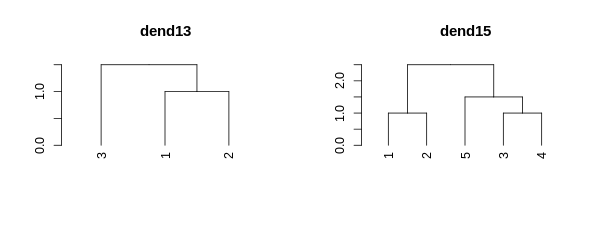Setting a dendrogram’s labels

We can get a vector with the tree’s labels:

# get the labels:
dend15 %>% labels
#>  1 2 5 3 4
# this is just like labels(dend)

Notice how the tree’s labels are not 1 to 5 by order, since the tree happened to place them in a different order. We can change the names of the labels:

# change the labels, and then print them:
dend15 %>% set("labels", c(111:115)) %>% labels
#>  111 112 113 114 115
# could also be done using:
# labels(dend) <- c(111:115)

We can change the type of labels to be characters. Not doing so may be a source of various bugs and problems in many functions.

dend15 %>% labels
#>  1 2 5 3 4
dend15 %>% set("labels_to_char") %>% labels
#>  "1" "2" "5" "3" "4"

We may also change their color and size:

par(mfrow = c(1,2))
dend15 %>% set("labels_col", "blue") %>% plot(main = "Change label's color") # change color
dend15 %>% set("labels_cex", 2) %>% plot(main = "Change label's size") # change color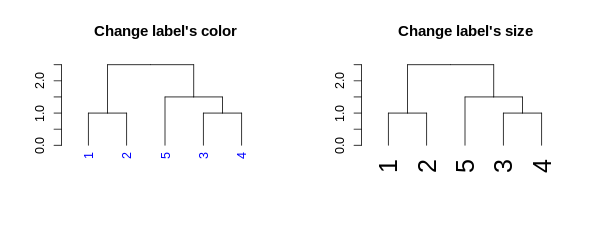The function recycles, from left to right, the vector of values we give it. We can use this to create more complex patterns:

# Produce a more complex dendrogram:
dend15_2 <- dend15 %>%
set("labels", c(111:115)) %>%    # change labels
set("labels_col", c(1,2,3)) %>%  # change color
set("labels_cex", c(2,1))        # change size

par(mfrow = c(1,2))
dend15 %>% plot(main = "Before")
dend15_2 %>% plot(main = "After")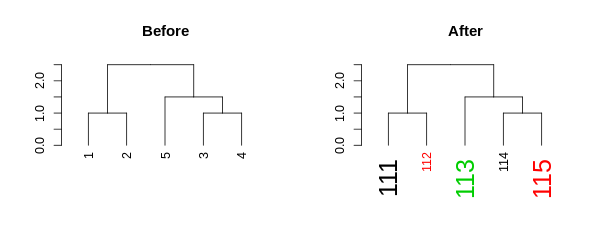Notice how these “labels parameters” are nested within the nodePar attribute:

# looking at only the left-most node of the "after tree":
dend15_2[][] %>% unclass %>% str
#>  int 1
#>  - attr(*, "label")= int 111
#>  - attr(*, "members")= int 1
#>  - attr(*, "height")= num 0
#>  - attr(*, "leaf")= logi TRUE
#>  - attr(*, "nodePar")=List of 3
#>   ..\$ lab.col: num 1
#>   ..\$ pch    : logi NA
#>   ..\$ lab.cex: num 2
# looking at only the nodePar attributes in this sub-tree:
dend15_2[][] %>% get_nodes_attr("nodePar")
#>         [,1]
#> lab.col 1
#> pch     NA
#> lab.cex 2

When it comes to color, we can also set the parameter “k”, which will cut the tree into k clusters, and assign a different color to each label (based on its cluster):

par(mfrow = c(1,2))
dend15 %>% set("labels_cex", 2) %>% set("labels_col", value = c(3,4)) %>%
plot(main = "Recycles color \nfrom left to right")
dend15 %>% set("labels_cex", 2) %>% set("labels_col", value = c(3,4), k=2) %>%
plot(main = "Color labels \nper cluster")
abline(h = 2, lty = 2)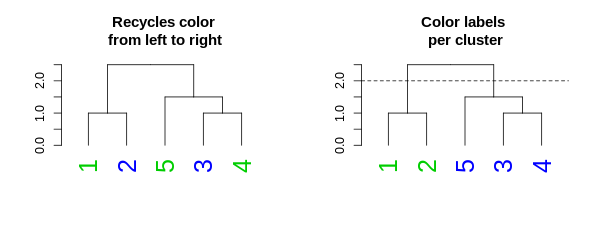Setting a dendrogram’s nodes/leaves (points)

Each node in a tree can be represented and controlled using the assign_values_to_nodes_nodePar, and for the special case of the nodes of leaves, the assign_values_to_leaves_nodePar function is more appropriate (and faster) to use. We can control the following properties: pch (point type), cex (point size), and col (point color). For example:

par(mfrow = c(2,3))
dend13 %>% set("nodes_pch", 19) %>% plot(main = "(1) Show the\n nodes (as a dot)") #1
dend13 %>% set("nodes_pch", 19) %>% set("nodes_cex", 2) %>%
plot(main = "(2) Show (larger)\n nodes") #2
dend13 %>% set("nodes_pch", 19) %>% set("nodes_cex", 2) %>% set("nodes_col", 3) %>%
plot(main = "(3) Show (larger+colored)\n nodes") #3

dend13 %>% set("leaves_pch", 19) %>% plot(main = "(4) Show the\n leaves (as a dot)") #4
dend13 %>% set("leaves_pch", 19) %>% set("leaves_cex", 2) %>%
plot(main = "(5) Show (larger)\n leaves") #5
dend13 %>% set("leaves_pch", 19) %>% set("leaves_cex", 2) %>% set("leaves_col", 3) %>%
plot(main = "(6) Show (larger+colored)\n leaves") #6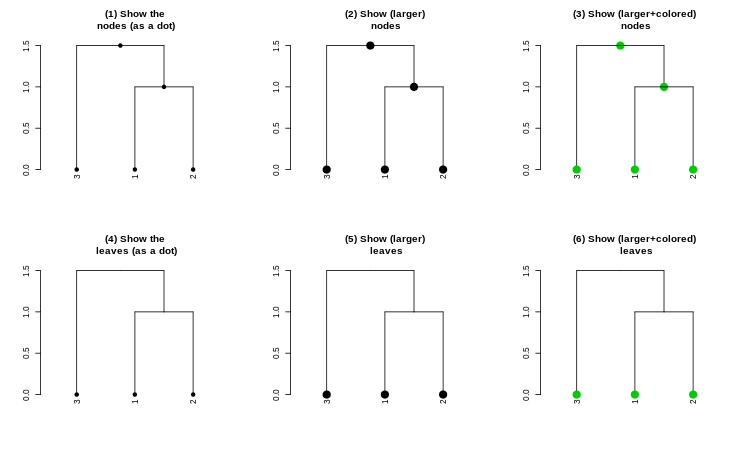And with recycling we can produce more complex outputs:

par(mfrow = c(1,2))
dend15 %>% set("nodes_pch", c(19,1,4)) %>% set("nodes_cex", c(2,1,2)) %>% set("nodes_col", c(3,4)) %>%
dend15 %>% set("leaves_pch", c(19,1,4)) %>% set("leaves_cex", c(2,1,2)) %>% set("leaves_col", c(3,4)) %>%
plot(main = "Adjust nodes\n(but only for leaves)")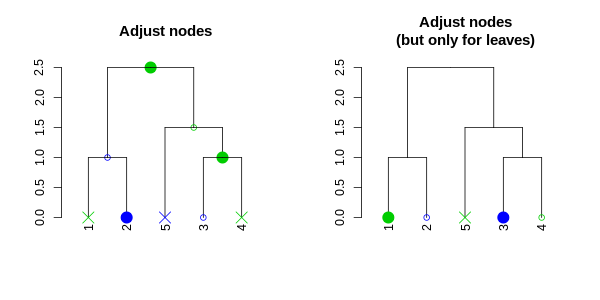Notice how recycling works in a depth-first order (which is just left to right, when we only adjust the leaves). Here are the node’s parameters after adjustment:

dend15 %>% set("nodes_pch", c(19,1,4)) %>%
set("nodes_cex", c(2,1,2)) %>% set("nodes_col", c(3,4)) %>% get_nodes_attr("nodePar")
#>     [,1] [,2] [,3] [,4] [,5] [,6] [,7] [,8] [,9]
#> pch 19   1    4    19   1    4    19   1    4
#> cex 2    1    2    2    1    2    2    1    2
#> col 3    4    3    4    3    4    3    4    3

We can also change the height of of the leaves by using the hang.dendrogram function:

par(mfrow = c(1,3))
dend13 %>% set("leaves_pch", 19) %>% set("leaves_cex", 2) %>% set("leaves_col", 2) %>% # adjust the leaves
hang.dendrogram %>% # hang the leaves
plot(main = "Hanging a tree")
dend13 %>% set("leaves_pch", 19) %>% set("leaves_cex", 2) %>% set("leaves_col", 2) %>% # adjust the leaves
hang.dendrogram(hang_height = .6) %>% # hang the leaves (at some height)
plot(main = "Hanging a tree (but lower)")
dend13 %>% set("leaves_pch", 19) %>% set("leaves_cex", 2) %>% set("leaves_col", 2) %>% # adjust the leaves
hang.dendrogram %>% # hang the leaves
hang.dendrogram(hang = -1) %>% # un-hanging the leaves
plot(main = "Not hanging a tree")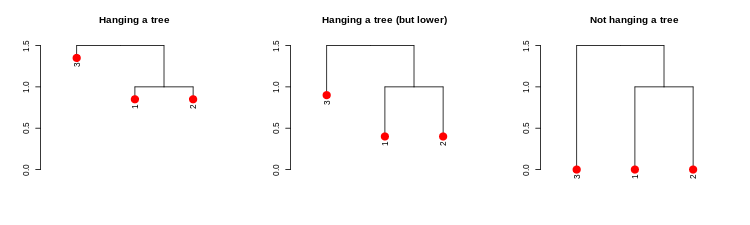An example of what this function does to the leaves heights:

dend13 %>% get_leaves_attr("height")
#>  0 0 0
dend13 %>% hang.dendrogram %>% get_leaves_attr("height")
#>  1.35 0.85 0.85

We can also control the general heights of nodes using raise.dendrogram:

par(mfrow = c(1,3))
dend13 %>% plot(main = "First tree", ylim = c(0,3))
dend13 %>%
raise.dendrogram (-1) %>%
plot(main = "One point lower", ylim = c(0,3))
dend13 %>%
raise.dendrogram (1) %>%
plot(main = "One point higher", ylim = c(0,3))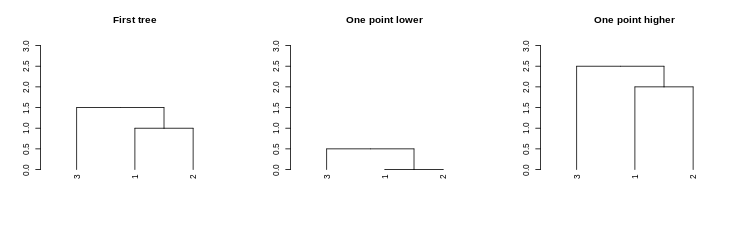If you wish to make the branches under the root have the same height, you can use the flatten.dendrogram function.

Setting a dendrogram’s branches

Similar to adjusting nodes, we can also control line width (lwd), line type (lty), and color (col) for branches:

par(mfrow = c(1,3))
dend13 %>% set("branches_lwd", 4) %>% plot(main = "Thick branches")
dend13 %>% set("branches_lty", 3) %>% plot(main = "Dashed branches")
dend13 %>% set("branches_col", 2) %>% plot(main = "Red branches")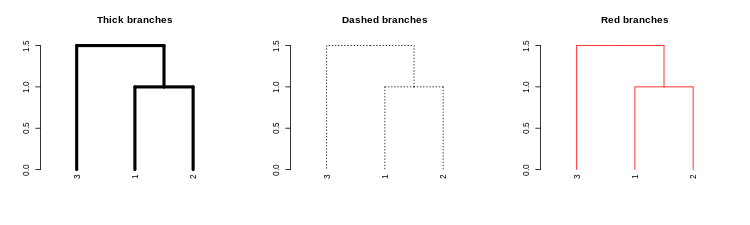We may also use recycling to create more complex patterns:

# Produce a more complex dendrogram:
dend15 %>%
set("branches_lwd", c(4,1)) %>%
set("branches_lty", c(1,1,3)) %>%
set("branches_col", c(1,2,3)) %>%
plot(main = "Complex branches", edge.root = TRUE)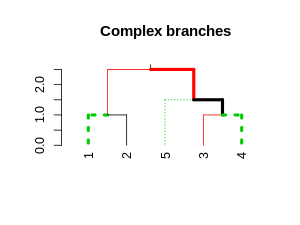Notice how the first branch (the root) is considered when going through and creating the tree, but it is ignored in the actual plotting (this is actually a “missing feature” in plot.dendrogram).

Coloring branches based on clustering

We may also control the colors of the branches based on using clustering:

par(mfrow = c(1,2))
dend15 %>% set("branches_k_color", k = 3) %>% plot(main = "Nice defaults")
dend15 %>% set("branches_k_color", value = 3:1, k = 3) %>%
plot(main = "Controlling branches' colors\n(via clustering)")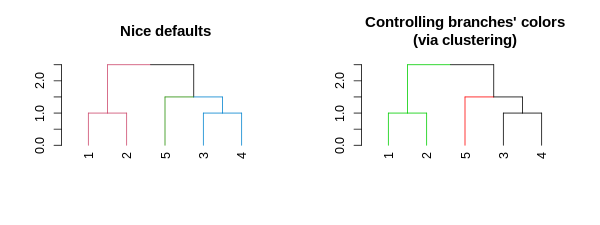# This is like using the `color_branches` function

The most powerful way to control branches is through the branches_attr_by_labels function (with variations through the set function). The function allows you to change col/lwd/lty of branches if they match some “labels condition”. Follow carefully:

par(mfrow = c(1,2))
dend15 %>% set("by_labels_branches_col", value = c(1,4)) %>%
plot(main = "Adjust the branch\n if ALL (default) of its\n labels are in the list")
dend15 %>% set("by_labels_branches_col", value = c(1,4), type = "any") %>%
plot(main = "Adjust the branch\n if ANY of its\n labels are in the list")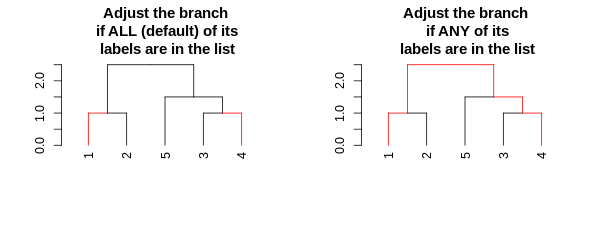We can use this to change the size/type/color of the branches:

# Using "Inf" in "TF_values" means to let the parameters stay as they are.
par(mfrow = c(1,3))
dend15 %>% set("by_labels_branches_col", value = c(1,4), TF_values = c(3,Inf)) %>%
plot(main = "Change colors")
dend15 %>% set("by_labels_branches_lwd", value = c(1,4), TF_values = c(8,1)) %>%
plot(main = "Change line width")
dend15 %>% set("by_labels_branches_lty", value = c(1,4), TF_values = c(3,Inf)) %>%
plot(main = "Change line type")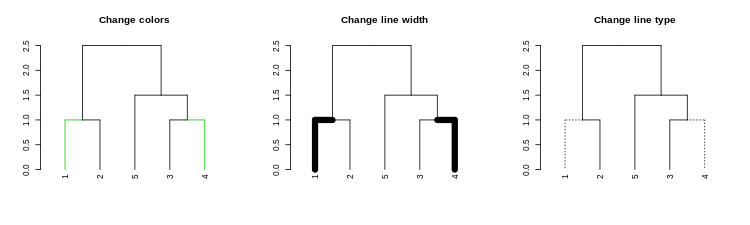Highlighting branches’ different heights using line width and color

The highlight_branches function helps to more easily see the topological structure of a tree, by adjusting branches appearence (color and line width) based on their height in the tree. For example:

dat <- iris[1:20,-5]
hca <- hclust(dist(dat))
hca2 <- hclust(dist(dat), method = "single")
dend <- as.dendrogram(hca)
dend2 <- as.dendrogram(hca2)

par(mfrow = c(1,3))
dend %>% highlight_branches_col %>% plot(main = "Coloring branches")
dend %>% highlight_branches_lwd %>% plot(main = "Emphasizing line-width")
dend %>% highlight_branches %>% plot(main = "Emphasizing color\n and line-width")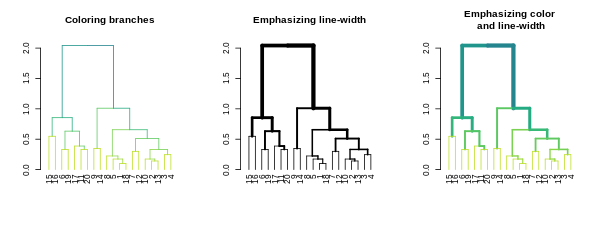Tanglegrams are even easier to compare when using

library(viridis)
par(mfrow = c(1,3))
dend %>% highlight_branches_col %>% plot(main = "Coloring branches \n (default is reversed viridis)")
dend %>% highlight_branches_col(viridis(100)) %>% plot(main = "It is better to use \n lighter colors in the leaves")
dend %>% highlight_branches_col(rev(magma(1000))) %>% plot(main = "The magma color pallatte\n is also good")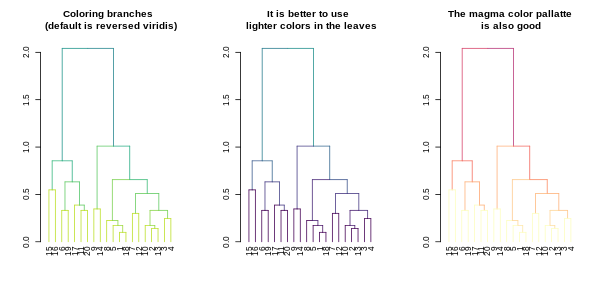dl <- dendlist(dend, dend2)
tanglegram(dl, sort = TRUE, common_subtrees_color_lines = FALSE, highlight_distinct_edges  = FALSE, highlight_branches_lwd = FALSE)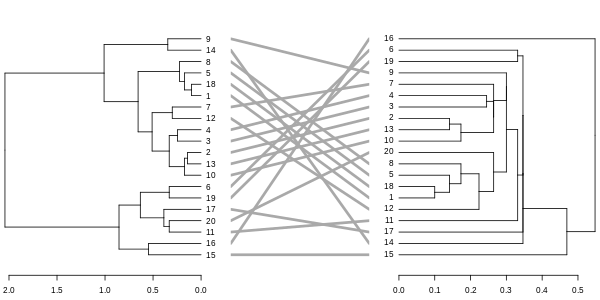tanglegram(dl)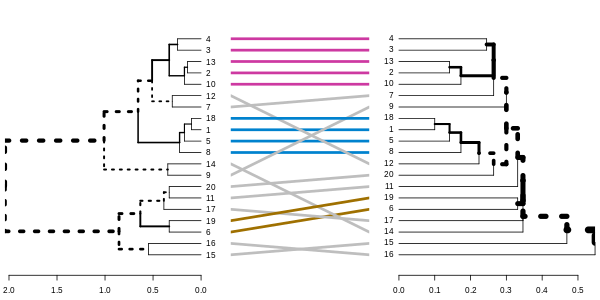tanglegram(dl, fast = TRUE)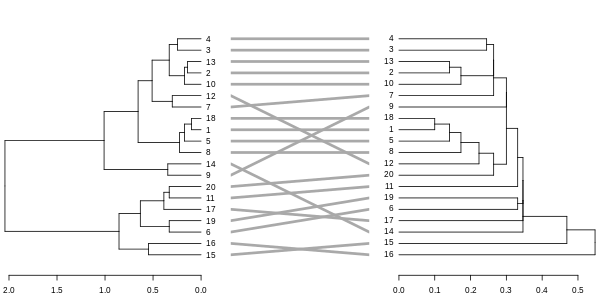dl <- dendlist(highlight_branches(dend), highlight_branches(dend2))
tanglegram(dl, sort = TRUE, common_subtrees_color_lines = FALSE, highlight_distinct_edges  = FALSE)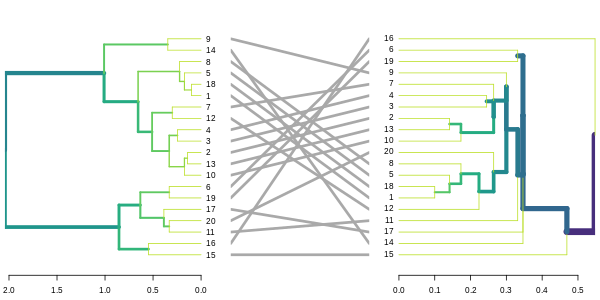# dend %>% set("highlight_branches_col") %>% plot

dl <- dendlist(dend, dend2) %>% set("highlight_branches_col")
tanglegram(dl, sort = TRUE, common_subtrees_color_lines = FALSE, highlight_distinct_edges  = FALSE)

Changing a dendrogram’s structure

Rotation

A dendrogram is an object which can be rotated on its hinges without changing its topology. Rotating a dendrogram in base R can be done using the reorder function. The problem with this function is that it is not very intuitive. For this reason the rotate function was written. It has two main arguments: the “object” (a dendrogram), and the “order” we wish to rotate it by. The “order” parameter can be either a numeric vector, used in a similar way we would order a simple character vector. Or, the order parameter can also be a character vector of the labels of the tree, given in the new desired order of the tree. It is also worth noting that some order are impossible to achieve for a given tree’s topology. In such cases, the function will do its “best” to get as close as possible to the requested rotation.

par(mfrow = c(1,3))
dend15 %>%
set("labels_colors") %>%
set("branches_k_color") %>%
plot(main = "First tree")
dend15 %>%
set("labels_colors") %>%
set("branches_k_color") %>%
rotate(as.character(5:1)) %>% #rotate to match labels new order
plot(main = "Rotated tree\n based on labels")
dend15 %>%
set("labels_colors") %>%
set("branches_k_color") %>%
rotate(5:1) %>% # the fifth label to go first is "4"
plot(main = "Rotated tree\n based on order")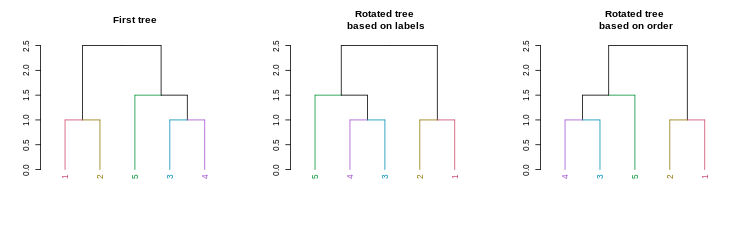A new convenience S3 function for sort (sort.dendrogram) was added:

dend110 <- c(1, 3:5, 7,9,10) %>% dist %>% hclust(method = "average") %>%
as.dendrogram %>% color_labels %>% color_branches

par(mfrow = c(1,3))
dend110 %>% plot(main = "Original tree")
dend110 %>% sort %>% plot(main = "labels sort")
dend110 %>% sort(type = "nodes") %>% plot(main = "nodes (ladderize) sort")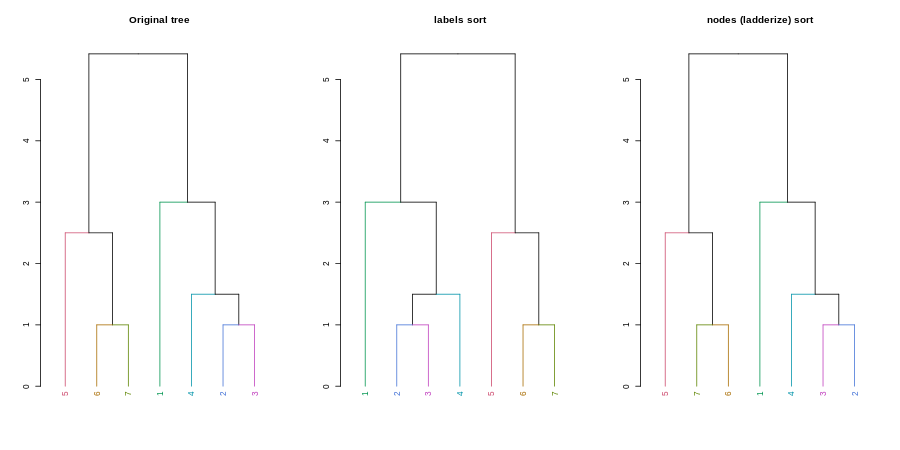Unbranching

We can unbranch a tree:

par(mfrow = c(1,3))
dend15 %>% plot(main = "First tree", ylim = c(0,3))
dend15 %>%
unbranch %>%
plot(main = "Unbranched tree", ylim = c(0,3))
dend15 %>%
unbranch(2) %>%
plot(main = "Unbranched tree (2)", ylim = c(0,3))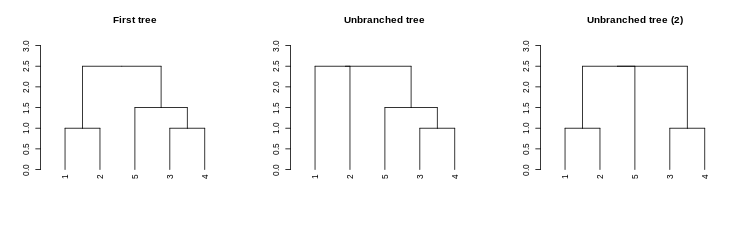Pruning

We can prune a tree based on the labels:

par(mfrow = c(1,2))
dend15 %>% set("labels_colors") %>%
plot(main = "First tree", ylim = c(0,3))
dend15 %>% set("labels_colors") %>%
prune(c("1","5")) %>%
plot(main = "Prunned tree", ylim = c(0,3))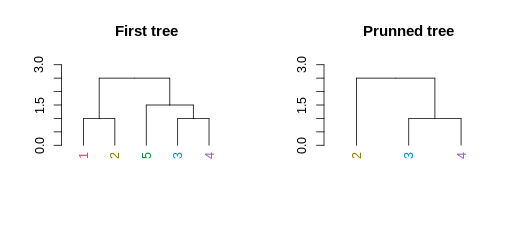For pruning two trees to have matching labels, we can use the intersect_trees function:

par(mfrow = c(1,2))
dend_intersected <- intersect_trees(dend13, dend15)
dend_intersected[] %>% plot
dend_intersected[] %>% plot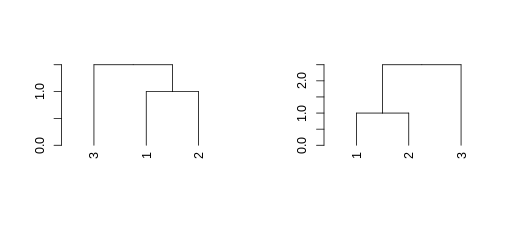Collapse branches

We can collapse branches under a tolerance level using the collapse_branch function:

# ladderize is like sort(..., type = "node")
dend <- iris[1:5,-5] %>% dist %>% hclust %>% as.dendrogram
par(mfrow = c(1,3))
dend %>% ladderize %>%  plot(horiz = TRUE); abline(v = .2, col = 2, lty = 2)
dend %>% collapse_branch(tol = 0.2) %>% ladderize %>% plot(horiz = TRUE)
dend %>% collapse_branch(tol = 0.2) %>% ladderize %>% hang.dendrogram(hang = 0) %>% plot(horiz = TRUE)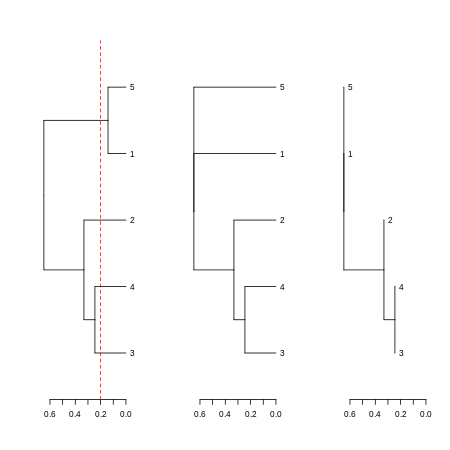Earlier we have seen how to highlight clusters in a dendrogram by coloring branches. We can also draw rectangles around the branches of a dendrogram in order to highlight the corresponding clusters. First the dendrogram is cut at a certain level, then a rectangle is drawn around selected branches. This is done using the rect.dendrogram, which is modeled based on the rect.hclust function. One advantage of rect.dendrogram over rect.hclust, is that it also works on horizontally plotted trees:

layout(t(c(1,1,1,2,2)))

dend15 %>% set("branches_k_color") %>% plot
dend15 %>% rect.dendrogram(k=3,
border = 8, lty = 5, lwd = 2)

dend15 %>% set("branches_k_color") %>% plot(horiz = TRUE)
dend15 %>% rect.dendrogram(k=3, horiz = TRUE,
border = 8, lty = 5, lwd = 2)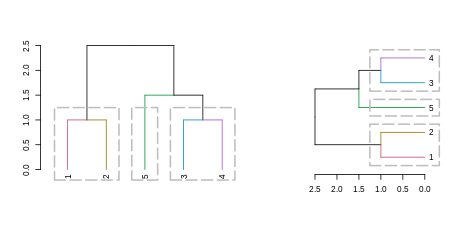Adding colored bars to a dendrogram may be useful to show clusters or some outside categorization of the items. For example:

is_odd <- ifelse(labels(dend15) %% 2, 2,3)
is_345 <- ifelse(labels(dend15) > 2, 3,4)
is_12 <- ifelse(labels(dend15) <= 2, 3,4)
k_3 <- cutree(dend15,k = 3, order_clusters_as_data = FALSE)
# The FALSE above makes sure we get the clusters in the order of the
# dendrogram, and not in that of the original data. It is like:
# cutree(dend15, k = 3)[order.dendrogram(dend15)]
the_bars <- cbind(is_odd, is_345, is_12, k_3)
the_bars[the_bars==2] <- 8

dend15 %>% plot
colored_bars(colors = the_bars, dend = dend15, sort_by_labels_order = FALSE)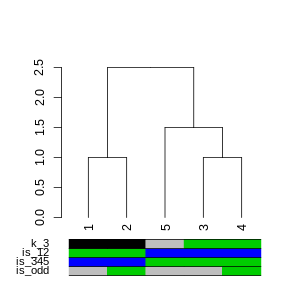# we use sort_by_labels_order = FALSE since "the_bars" were set based on the
# labels order. The more common use case is when the bars are based on a second variable
# from the same data.frame as dend was created from. Thus, the default
# sort_by_labels_order = TRUE would make more sense.

Another example, based on mtcars (in which the default of sort_by_labels_order = TRUE makes sense):

dend_mtcars <- mtcars[, c("mpg", "disp")] %>% dist %>% hclust(method = "average") %>% as.dendrogram

par(mar = c(10,2,1,1))
plot(dend_mtcars)
the_bars <- ifelse(mtcars\$am, "grey", "gold")
colored_bars(colors = the_bars, dend = dend_mtcars, rowLabels = "am")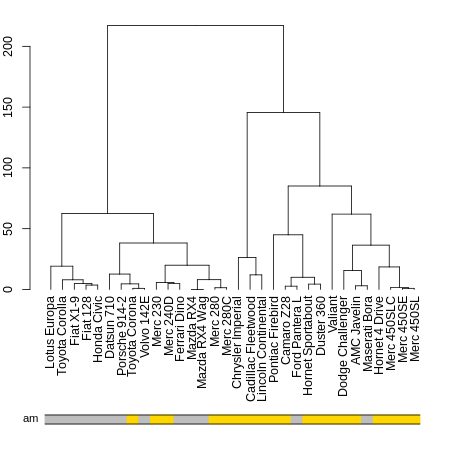ggplot2 integration

The core process is to transform a dendrogram into a ggdend object using as.ggdend, and then plot it using ggplot (a new S3 ggplot.ggdend function is available). These two steps can be done in one command with either the function ggplot or ggdend.

The reason we want to have as.ggdend (and not only ggplot.dendrogram), is (1) so that you could create your own mapping of ggdend and, (2) since as.ggdend might be slow for large trees, it is probably better to be able to run it only once for such cases.

A ggdend class object is a list with 3 components: segments, labels, nodes. Each one contains the graphical parameters from the original dendrogram, but in a tabular form that can be used by ggplot2+geom_segment+geom_text to create a dendrogram plot.

The function prepare.ggdend is used by plot.ggdend to take the ggdend object and prepare it for plotting. This is because the defaults of various parameters in dendrogram’s are not always stored in the object itself, but are built-in into the plot.dendrogram function. For example, the color of the labels is not (by default) specified in the dendrogram (only if we change it from black to something else). Hence, when taking the object into a different plotting engine (say ggplot2), we want to prepare the object by filling-in various defaults. This function is automatically invoked within the plot.ggdend function. You would probably use it only if you’d wish to build your own ggplot2 mapping.

# Create a complex dend:
dend <- iris[1:30,-5] %>% dist %>% hclust %>% as.dendrogram %>%
set("branches_k_color", k=3) %>% set("branches_lwd", c(1.5,1,1.5)) %>%
set("branches_lty", c(1,1,3,1,1,2)) %>%
set("labels_colors") %>% set("labels_cex", c(.9,1.2)) %>%
set("nodes_pch", 19) %>% set("nodes_col", c("orange", "black", "plum", NA))
# plot the dend in usual "base" plotting engine:
plot(dend)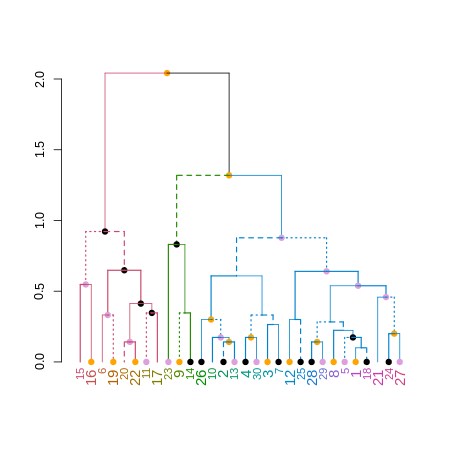# Now let's do it in ggplot2 :)
ggd1 <- as.ggdend(dend)
library(ggplot2)
# the nodes are not implemented yet.
ggplot(ggd1) # reproducing the above plot in ggplot2 :)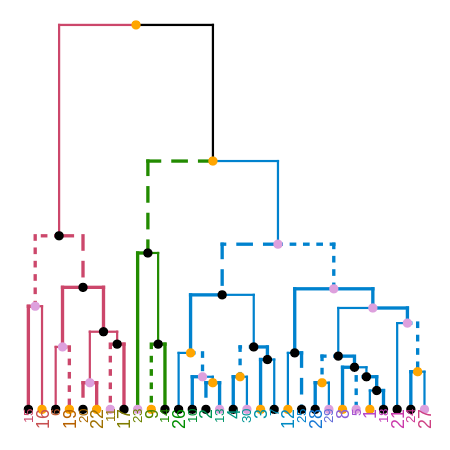ggplot(ggd1, horiz = TRUE, theme = NULL) # horiz plot (and let's remove theme) in ggplot2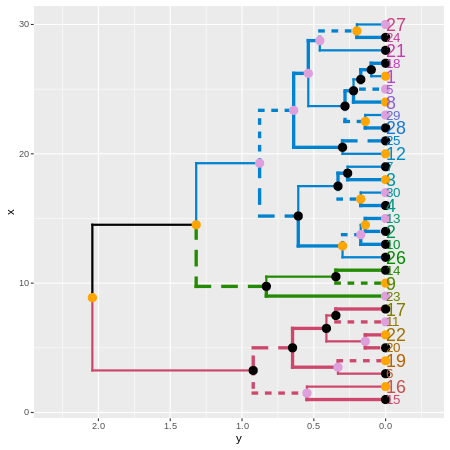# Adding some extra spice to it...
# ggplot(ggd1) + scale_y_reverse(expand = c(0.2, 0)) + coord_polar(theta="x")
# The text doesn't look so great, so let's remove it:
ggplot(ggd1, labels = FALSE) + scale_y_reverse(expand = c(0.2, 0)) + coord_polar(theta="x")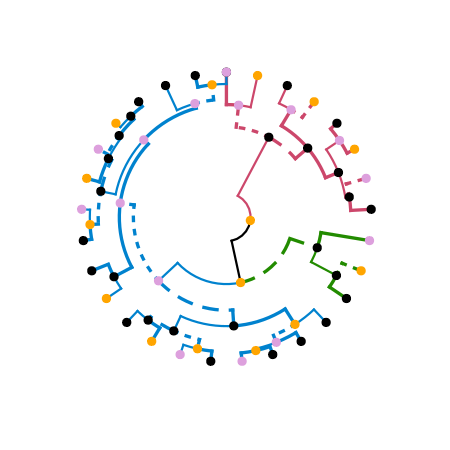Credit: These functions are extended versions of the functions ggdendrogram, dendro_data (and the hidden dendrogram_data) from Andrie de Vries’s ggdendro package. The motivation for this fork is the need to add more graphical parameters to the plotted tree. This required a strong mixture of functions from ggdendro and dendextend (to the point that it seemed better to just fork the code into its current form).

Enhancing other packages

The dendextend package aims to extend and enhance features from the R ecosystem. Let us take a look at several examples.

DendSer

The DendSer package helps in re-arranging a dendrogram to optimize visualization-based cost functions. Until now it was only used for hclust objects, but it can easily be connected to dendrogram objects by trying to turn the dendrogram into hclust, on which it runs DendSer. This can be used to rotate the dendrogram easily by using the rotate_DendSer function:

if(require(DendSer)) {
par(mfrow = c(1,2))
DendSer.dendrogram(dend15)

dend15 %>% color_branches %>%                      plot
dend15 %>% color_branches %>% rotate_DendSer %>%   plot
}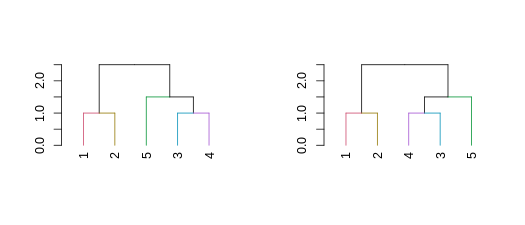gplots

The gplots package brings us the heatmap.2 function. In it, we can use our modified dendrograms to get more informative heat-maps:

library(gplots)

x  <- as.matrix(datasets::mtcars)

heatmap.2(x)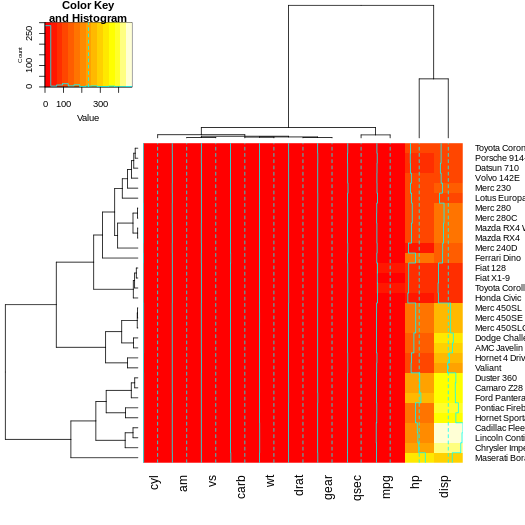# now let's spice up the dendrograms a bit:
Rowv  <- x %>% dist %>% hclust %>% as.dendrogram %>%
set("branches_k_color", k = 3) %>% set("branches_lwd", 4) %>%
#    rotate_DendSer(ser_weight = dist(x))
Colv  <- x %>% t %>% dist %>% hclust %>% as.dendrogram %>%
set("branches_k_color", k = 2) %>% set("branches_lwd", 4) %>%
#    rotate_DendSer(ser_weight = dist(t(x)))

heatmap.2(x, Rowv = Rowv, Colv = Colv)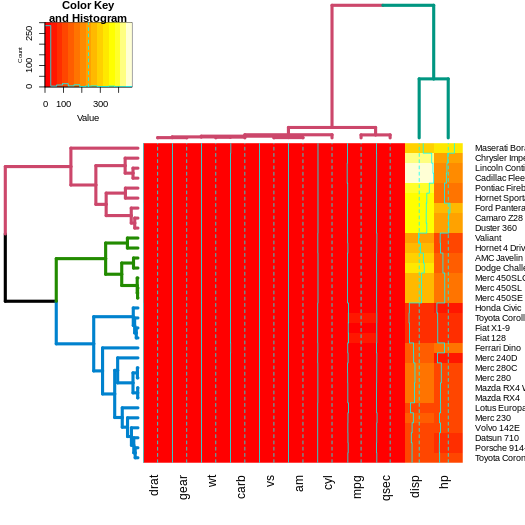NMF

The same as gplots, NMF offers a heatmap function called aheatmap. We can update it just as we would heatmap.2.

Since NMF was removed from CRAN (it could still be installed from source), the example code is still available but not ran in this vignette.

# library(NMF)
#
# x  <- as.matrix(datasets::mtcars)
#
# # now let's spice up the dendrograms a bit:
# Rowv  <- x %>% dist %>% hclust %>% as.dendrogram %>%
#    set("branches_k_color", k = 3) %>% set("branches_lwd", 4) %>%
# #    rotate_DendSer(ser_weight = dist(x))
# Colv  <- x %>% t %>% dist %>% hclust %>% as.dendrogram %>%
#    set("branches_k_color", k = 2) %>% set("branches_lwd", 4) %>%
# #    rotate_DendSer(ser_weight = dist(t(x)))
#
# aheatmap(x, Rowv = Rowv, Colv = Colv)

heatmaply

The heatmaply package create interactive heat-maps that are usable from the R console, in the ‘RStudio’ viewer pane, in ‘R Markdown’ documents, and in ‘Shiny’ apps. By hovering the mouse pointer over a cell or a dendrogram to show details, drag a rectangle to zoom.

The use is very similar to what we’ve seen before, we just use heatmaply instead of heatmap.2:

x  <- as.matrix(datasets::mtcars)
# heatmaply(x)
# now let's spice up the dendrograms a bit:
Rowv  <- x %>% dist %>% hclust %>% as.dendrogram %>%
set("branches_k_color", k = 3) %>% set("branches_lwd", 4) %>%
#    rotate_DendSer(ser_weight = dist(x))
Colv  <- x %>% t %>% dist %>% hclust %>% as.dendrogram %>%
set("branches_k_color", k = 2) %>% set("branches_lwd", 4) %>%
#    rotate_DendSer(ser_weight = dist(t(x)))

Here we need to use cache=FALSe in the markdown:

library(heatmaply)
heatmaply(x, Rowv = Rowv, Colv = Colv)

I avoided running the code from above due to space issues on CRAN. For live examples, please go to:

dynamicTreeCut

The cutreeDynamic function offers a wrapper for two methods of adaptive branch pruning of hierarchical clustering dendrograms. The results of which can now be visualized by both updating the branches, as well as using the colored_bars function (which was adjusted for use with plots of dendrograms):

# let's get the clusters
library(dynamicTreeCut)
data(iris)
x  <- iris[,-5] %>% as.matrix
hc <- x %>% dist %>% hclust
dend <- hc %>% as.dendrogram

# Find special clusters:
clusters <- cutreeDynamic(hc, distM = as.matrix(dist(x)), method = "tree")
# we need to sort them to the order of the dendrogram:
clusters <- clusters[order.dendrogram(dend)]
clusters_numbers <- unique(clusters) - (0 %in% clusters)
n_clusters <- length(clusters_numbers)

library(colorspace)
cols <- rainbow_hcl(n_clusters)
true_species_cols <- rainbow_hcl(3)[as.numeric(iris[,][order.dendrogram(dend),5])]
dend2 <- dend %>%
branches_attr_by_clusters(clusters, values = cols) %>%
color_labels(col =   true_species_cols)
plot(dend2)
clusters <- factor(clusters)
levels(clusters)[-1]  <- cols[-5][c(1,4,2,3)]
# Get the clusters to have proper colors.
# fix the order of the colors to match the branches.
colored_bars(clusters, dend, sort_by_labels_order = FALSE)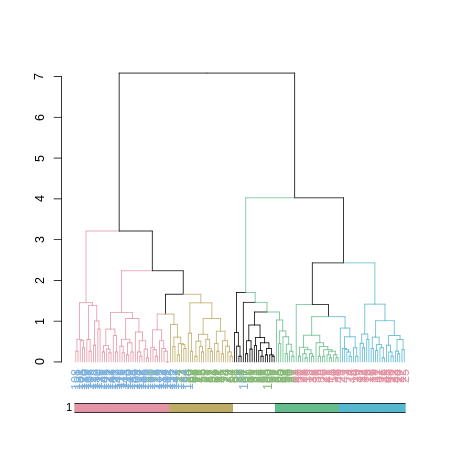# here we used sort_by_labels_order = FALSE since the clusters were already sorted based on the dendrogram's order

pvclust

The pvclust library calculates “p-values”" for hierarchical clustering via multiscale bootstrap re-sampling. Hierarchical clustering is done for given data and p-values are computed for each of the clusters. The dendextend package let’s us reproduce the plot from pvclust, but with a dendrogram (instead of an hclust object), which also lets us extend the visualization.

par(mfrow = c(1,2))

library(pvclust)
data(lung) # 916 genes for 73 subjects
set.seed(13134)
result <- pvclust(lung[1:100, 1:10],
method.dist="cor", method.hclust="average", nboot=10)

# with pvrect
plot(result)
pvrect(result)

# with a dendrogram of pvrect
dend <- as.dendrogram(result)
result %>% as.dendrogram %>%
plot(main = "Cluster dendrogram with AU/BP values (%)\n reproduced plot with dendrogram")
result %>% text
result %>% pvrect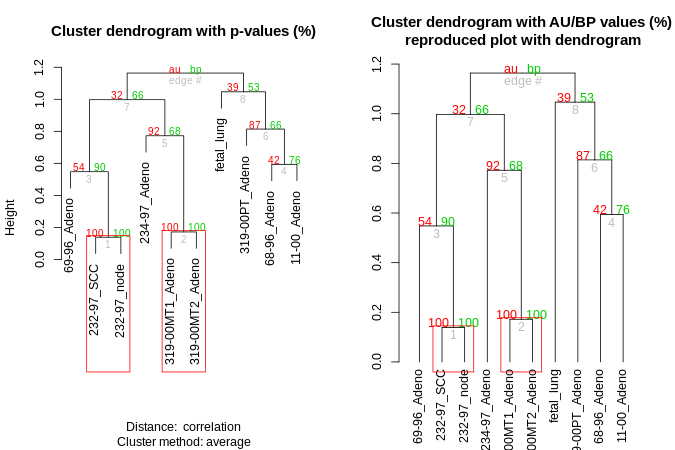Let’s color and thicken the branches based on the p-values:

par(mfrow = c(2,2))

# with a modified dendrogram of pvrect
dend %>% pvclust_show_signif(result) %>%
plot(main = "Cluster dendrogram \n bp values are highlighted by signif")

dend %>% pvclust_show_signif(result, show_type = "lwd") %>%
plot(main = "Cluster dendrogram with AU/BP values (%)\n bp values are highlighted by signif")
result %>% text
result %>% pvrect(alpha=0.95)

plot(main = "Cluster dendrogram with AU/BP values (%)\n bp values are colored by signif")

dend %>%
pvclust_show_signif(result) %>%
plot(main = "Cluster dendrogram with AU/BP values (%)\n bp values are colored+highlighted by signif")
result %>% text
result %>% pvrect(alpha=0.95)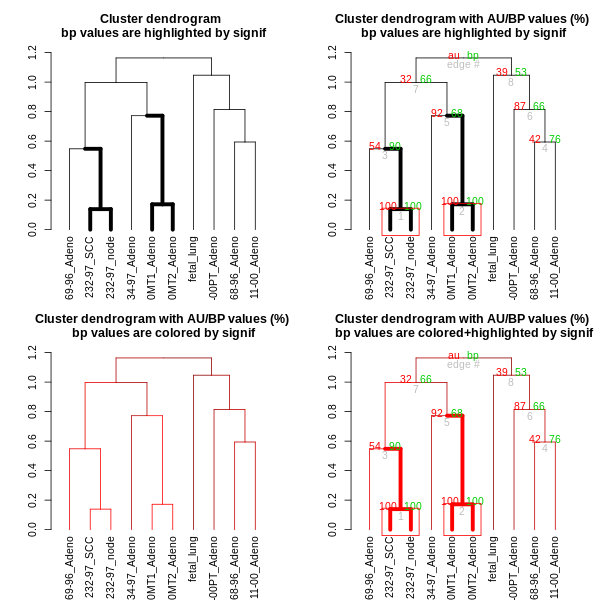circlize

Circular layout is an efficient way for the visualization of huge amounts of information. The circlize package provides an implementation of circular layout generation in R, including a solution for dendrogram objects produced using dendextend:

library(circlize)

dend <- iris[1:40,-5] %>% dist %>% hclust %>% as.dendrogram %>%
set("branches_k_color", k=3) %>% set("branches_lwd", c(5,2,1.5)) %>%
set("branches_lty", c(1,1,3,1,1,2)) %>%
set("labels_colors") %>% set("labels_cex", c(.6,1.5)) %>%
set("nodes_pch", 19) %>% set("nodes_col", c("orange", "black", "plum", NA))

par(mar = rep(0,4))
circlize_dendrogram(dend)